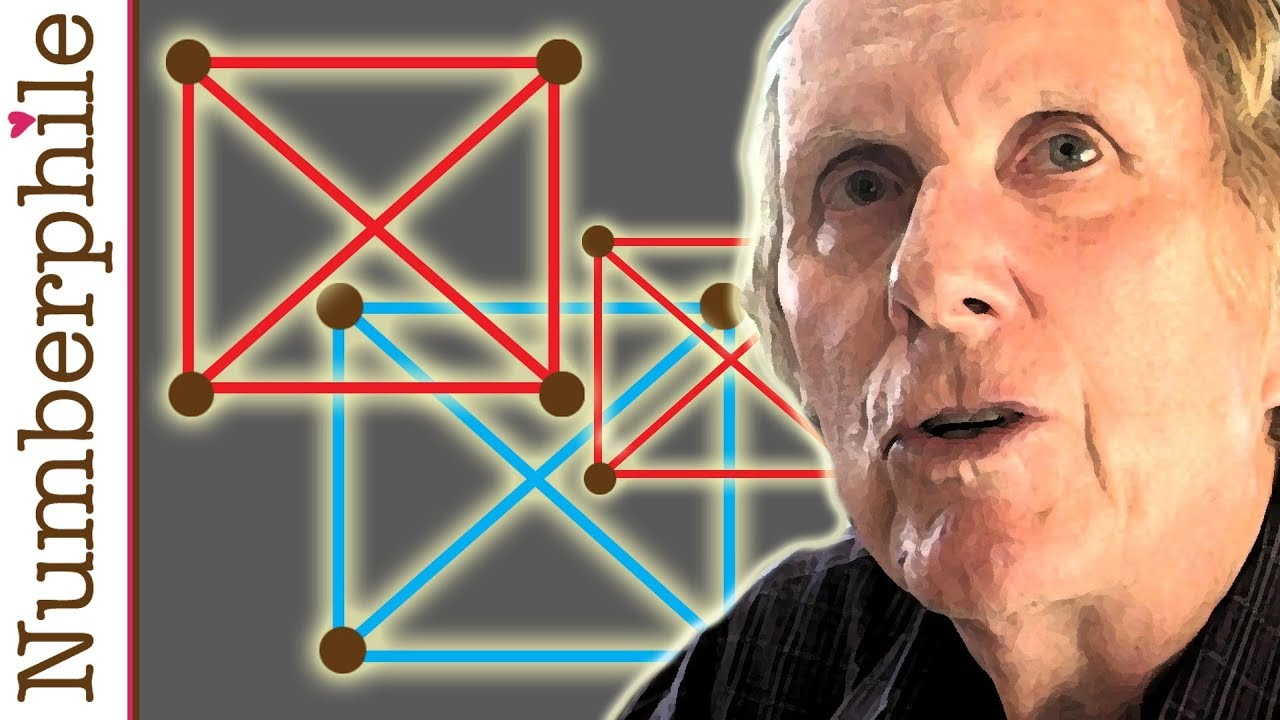Turn your brain into black hole

Hi,
here it goes, here it comes! Today I want to write about Graham’s number which is very interesting number and highest number which has some usage in mathematics.

Ok first of all, what is its purpose?
Imagine square where all vertices are connected. You can connect them by two colors, blue and red. You are trying to avoid one special pattern whichyou can see behing the mathematician Ron Graham who invented this number about which I will talk in a while. So those points can not be connected by single color the thing is that when you increase the dimension it is little harder (you already now everything about dimensions if you read my last post).When you have 3rd dimension as you can see on the right there are 8 vertices.
This means that there are 28 lines between those dots.
Yes it is possible to avoid the special configuration. When you continue to fourth dimension you can still do it but here comes the problem. In fourth dimension there are 2^120 possible ways to color this cube. This is such a large number that no computer can solve it, in decent amount of time. Now you need to make some better way to solve it. Through some better way which I dont know mathematicians got up to 13th dimension, now they dont know maybe it is possible maybe not but the number when we are COMPLETELY sure that it is not possible is Graham’s number.

To show you how large is Graham’s number I first of all have to explain Knuth’s up-arrow notation.
3+3+3=3*3
3*3*3=3^3
3^3=3↑3=27
That’s about it when you have one arrow. It gets better when you have two:
3↑↑2=3^3=27 , easy.
3↑↑3=3^3^3=3^27=over 7 trillions$\begin{matrix} a\uparrow\uparrow b & = {\ ^{b}a} = & \underbrace{a^{a^{{}^{.\,^{.\,^{.\,^a}}}}}} & = & \underbrace{a\uparrow (a\uparrow(\dots\uparrow a))} \\ & & b\mbox{ copies of }a & & b\mbox{ copies of }a \end{matrix}$
Lets see three of them:
2↑↑↑2=2↑↑(2↑↑2)=2↑↑4=2^2^2^2=2^2^4=2^16=65536
2↑↑↑3=2↑↑(2↑↑(2↑↑2)=2↑↑65536$\begin{matrix} a\uparrow\uparrow\uparrow b= & \underbrace{a_{}\uparrow\uparrow (a\uparrow\uparrow(\dots\uparrow\uparrow a))}\\ & b\mbox{ copies of }a \end{matrix}$
Easy it continues and it gets hella huge.
So when I found out that Graham’s number needs arrow notation to be written I was like: “Easy, there will be few arrows, arrow notation is such extreme tool.”
Then after I found out how big is Graham’s number I was just like: “Oops!” You will se why.
So lets define the number g1
g1=3↑↑↑↑3
=3↑↑↑(3↑↑↑3)
=3↑↑↑(3↑↑(3↑↑3)

=3↑↑↑(3↑↑(3↑(3↑3)
=3↑↑↑(3↑↑(3↑27) i think that this is enough for ilustration.
now lets define g2=3↑↑↑↑↑↑↑…3 number of arrows equals to g1

Surprised? We are not even in beginning.
g3=3↑↑↑↑…3 number of arrows equals to g2 … so you know the process, this continues up to g64 which is Graham’s number.

Ok this is extremely large number and to remind you it is number of dimensions, which means that the combinations are even much higher, not kidding.
The Graham’s number has real meaning but I am not really able to understand so if you want to check it, somewhere in the video Ron Graham explains it. Also Numberphile has some other videos about it so check them out.
Next time I will write about Ackermann function. After that you will get this XKCD joke: